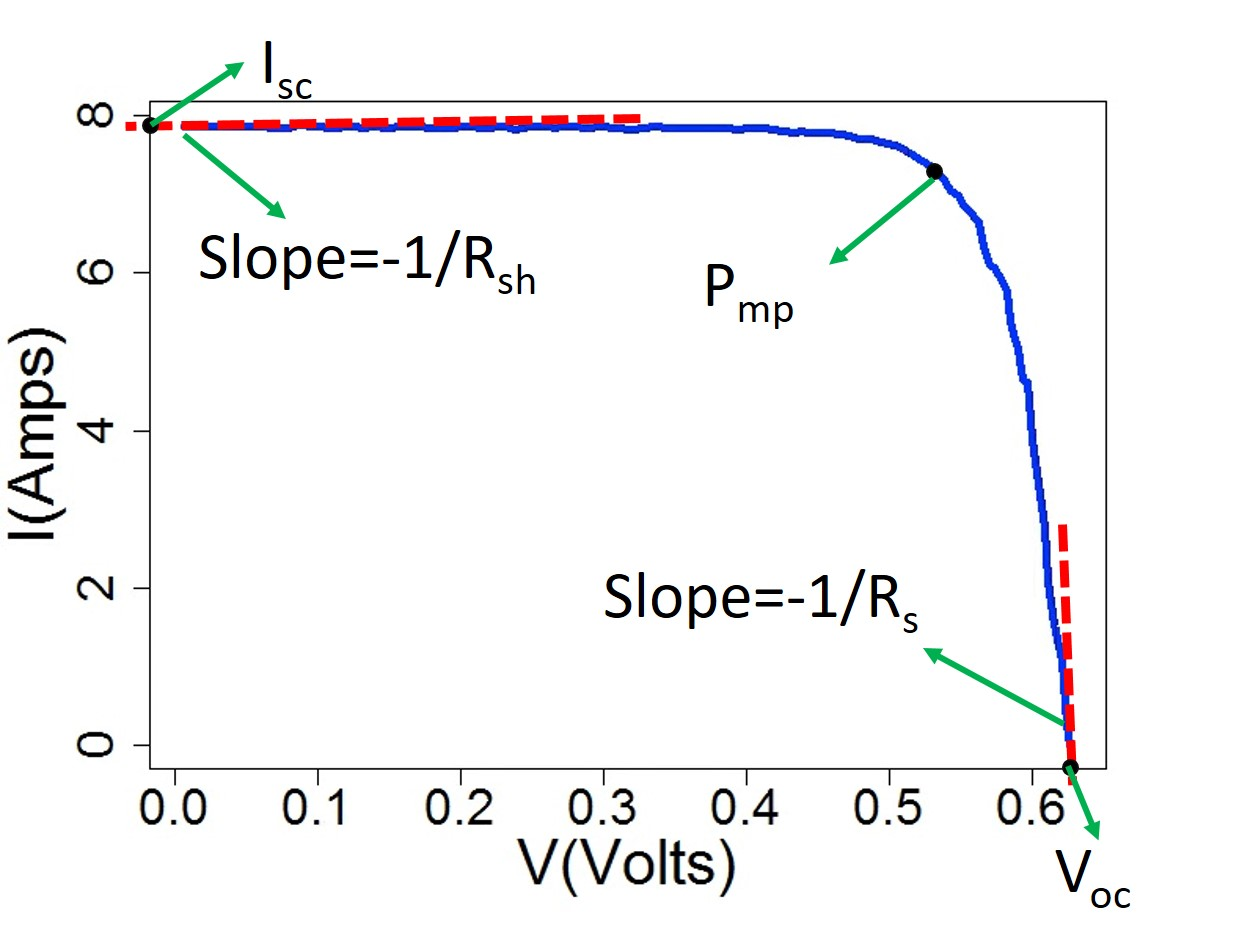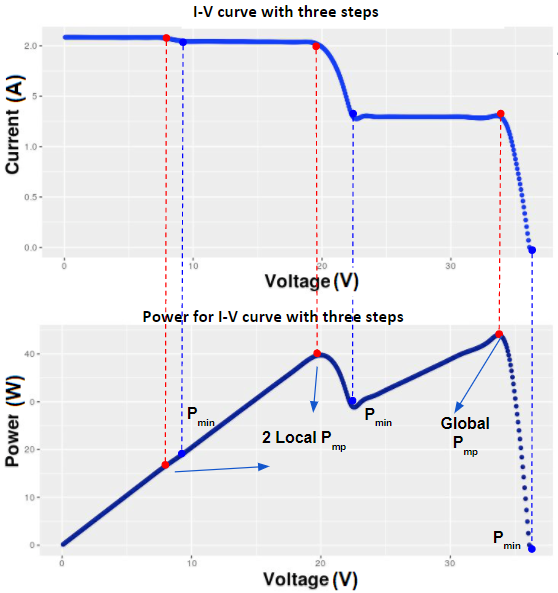# Introduction to $$I-V$$ Curves

#### 2021-04-14

An $$I-V$$ curve indicates the relationship between current and voltage for a solar cell or module. Thus for a single $$I-V$$ curve, the dataset usually consists of several data points of voltage $$V$$ and the associated current $$I$$. As is shown in Figure 1, a standard $$I-V$$ curve has the shape of a concave curve with nearly no change of current at small voltage, and a sharp decrease of current at a certain voltage point.

Solar cell parameters in $$I-V$$ curves are important in evaluating the performance and degradation of PV modules. These performance parameters include the maximum power point $$P_{mp}$$, short-circuit current $$I_{sc}$$, open-circuit voltage $$V_{oc}$$, shunt resistance $$R_{sh}$$, series resistance $$R_s$$, and fill factor $$FF$$. The first five of these parameters are illustrated in Figure 1. $$I_{sc}$$ is defined as the current at zero voltage (the y-intercept of the $$I-V$$ curve), while $$V_{oc}$$ is the voltage at zero current (the x-intercept). $$R_{sh}$$ is equivalent to the negative of inverse slope of the $$I-V$$ curve near $$I_{sc}$$. $$R_s$$ is equivalent to the negative of inverse slope of the $$I-V$$ curve near $$V_{oc}$$. $$P_{mp}$$ is the maximum product of current and voltage on the $$I-V$$ curve. $$FF$$ is defined as the ratio of the maximum power from the solar cell to the product of $$V_{oc}$$ and $$I_{sc}$$, it measures the “squareness” of the solar cell. $$FF$$ is not shown in Figure 1 directly, but can be calculated with the equation

$\begin{equation} FF= \frac{P_{max}}{I_{sc}*V_{oc}} \label{ff} \end{equation}$Figure 1: A standard $$I-V$$ curve and $$I-V$$ features. $$I-V$$ curve shows the relationship between current($$I$$) and voltage ($$V$$). $$I-V$$ features are maximum power point ($$P_{mp}$$), short-circuit current ($$I_{sc}$$), open-circuit voltage ($$V_{oc}$$), shunt resistance ($$R_{sh}$$), series resistance ($$R_s$$), and fill factor ($$FF$$)

We define the in the $$I-V$$ curves as how many typical $$I-V$$ curve shapes appear in the current-voltage relationship. The standard $$I-V$$ curve shown in Figure 1 is said to have only one step. There are cases (e.g. when it is cloudy) where several steps are present in a single $$I-V$$ curve due to activation of the bypass diodes. An example of $$I-V$$ curves with steps is demonstrated in Figure 2. This $$I-V$$ curve looks like a combination of three standard $$I-V$$ curves. This pattern of $$I-V$$ curves is an indication of mismatch between different areas of the array of module under test. This may be caused by a partial shading of the PV array or damage of PV cells, causing bypass diodes to activate. If a step is caused by partially shaded array, then the step would be transient and disappear from future $$I-V$$ curves. However, if the PV cell is damaged, then the step would be permanent.Figure 2: An example of $$I-V$$ curve that has three steps. The $$I-V$$ curve is a combination of three standard $$I-V$$ curves. There are three local maximum power point for each standard $$I-V$$ curves. Out of these three, one is the global maximum power point.

## Load data and run code to extract $$I-V$$ features

library(ddiv)
## Use the example IV curve data that has two steps
## Load the IV curve data set
data(IV_step2)
IV2 <- data.frame(IV_step2)
#?IV_step2

## Calculate number of steps in IV curve
IVsteps(IV2$I,IV2$V,plot.option=FALSE)
## Warning in id.psi.in & id.psi.far: longer object length is not a multiple of
## shorter object length

## Warning in id.psi.in & id.psi.far: longer object length is not a multiple of
## shorter object length

## Warning in id.psi.in & id.psi.far: longer object length is not a multiple of
## shorter object length

## Warning in id.psi.in & id.psi.far: longer object length is not a multiple of
## shorter object length
## $step ##  2 ## ##$xsep
##       V1
## 1 10.594
## Extract two sets of IV features for each sub IV curves
IVExtractResult(IV2,plot.option=FALSE)
## Warning in id.psi.in & id.psi.far: longer object length is not a multiple of
## shorter object length

## Warning in id.psi.in & id.psi.far: longer object length is not a multiple of
## shorter object length

## Warning in id.psi.in & id.psi.far: longer object length is not a multiple of
## shorter object length

## Warning in id.psi.in & id.psi.far: longer object length is not a multiple of
## shorter object length
##   step            Isc                Rsh              Voc              Rs
## 1    2 V1#1.732#1.917 V1#2732.708#46.831 V1#68.612#37.133 V1#34.319#1.082
##                Pmp            Imp              Vmp             FF    Cutoff
## 1 V1#17.841#55.137 V1#1.692#1.646 V1#10.544#33.489 V1#15.01#77.46 V1#10.594